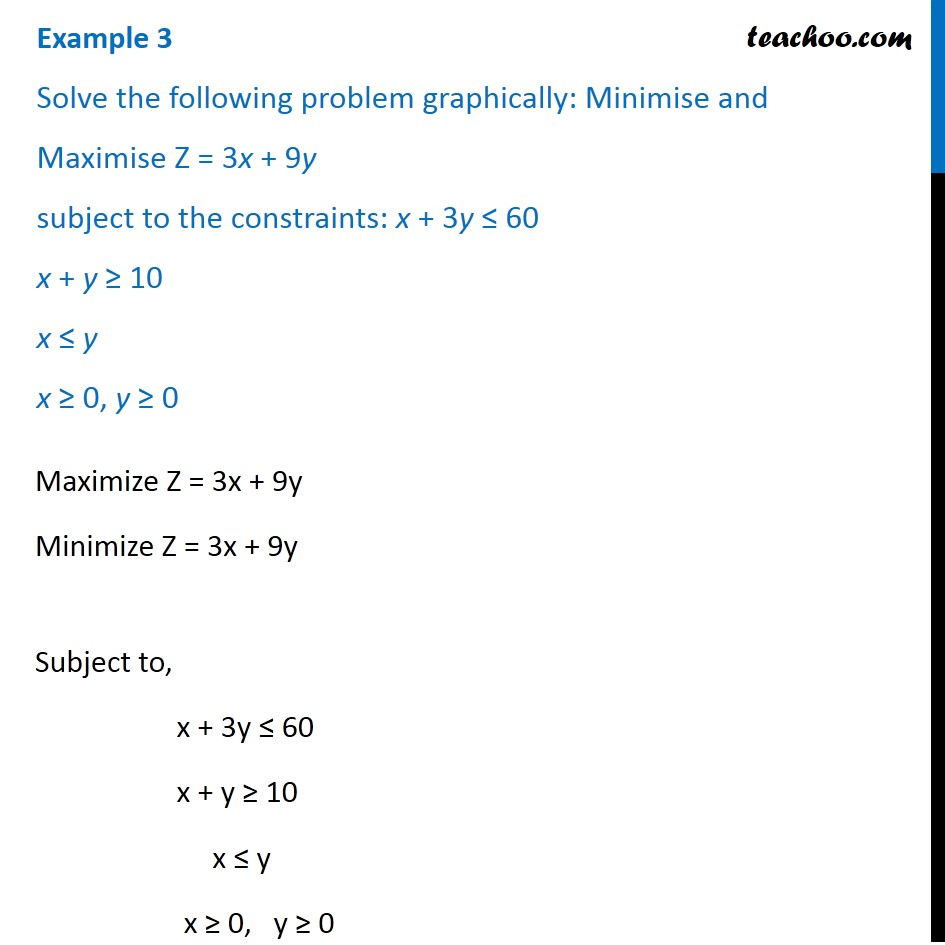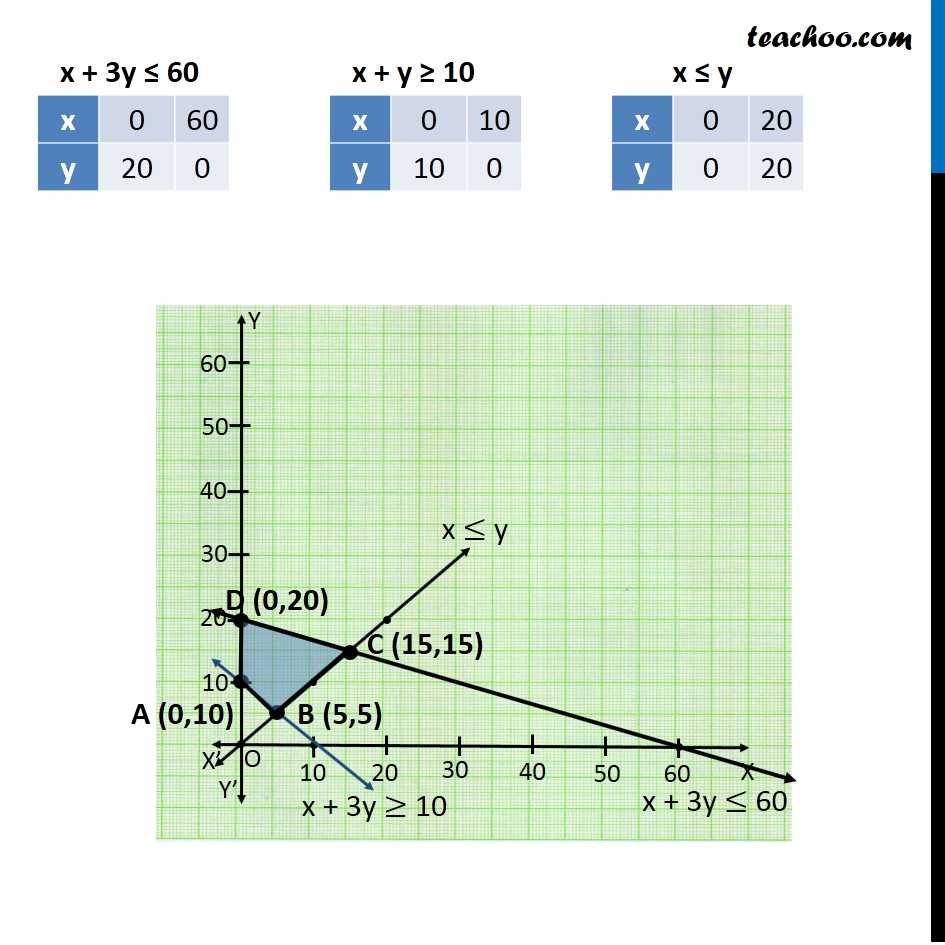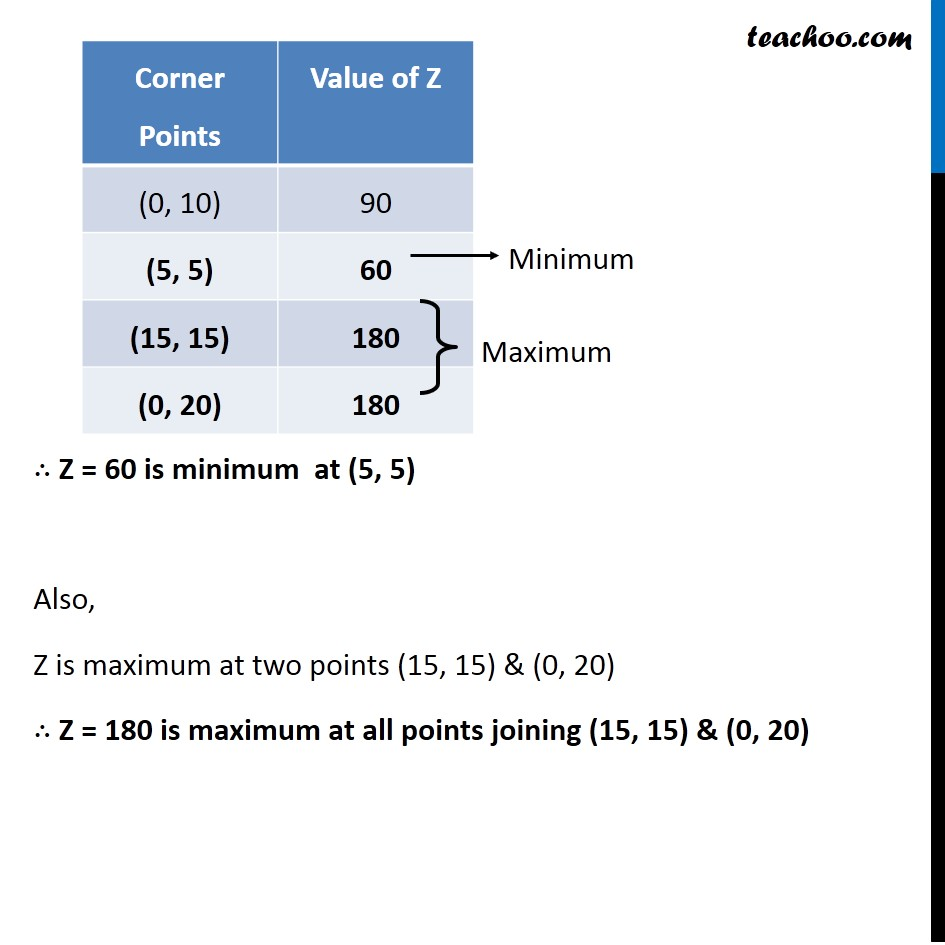Linear equations given - Bounded

Chapter 12 Class 12 Linear Programming
Concept wiseLearn in your speed, with individual attention - Teachoo Maths 1-on-1 Class

### Transcript

Example 3 Solve the following problem graphically: Minimise and Maximise Z = 3x + 9y subject to the constraints: x + 3y ≤ 60 x + y ≥ 10 x ≤ y x ≥ 0, y ≥ 0 Maximize Z = 3x + 9y Minimize Z = 3x + 9y Subject to, x + 3y ≤ 60 x + y ≥ 10 x ≤ y x ≥ 0, y ≥ 0 ∴ Z = 60 is minimum at (5, 5) Also, Z is maximum at two points (15, 15) & (0, 20) ∴ Z = 180 is maximum at all points joining (15, 15) & (0, 20)